# Visualizing Optimization in Linear Regression and Logistic Regression

This is one post in a series for machine learning optimization animations. Each plot can serve as a flashcard for easy consumption.Flying over Town Beach near the tip of Long Island, Spring 2019. Picture taken by me with DJI Mavic Air.

If you are like me, you may prefer looking at pictures that move to pages of Greek symbols when it comes to learning math. It’s more intuitive, more fun, and a great way to look under the hood and debug if things go wrong. So here I’m not going to bore you with equations. Equations and long derivations are important, but you already have countless books and notes for them.

I believe animation is the best way to learn, review and internalize math for both beginners and long-term practitioners. One of my favorite people in this area is Grant Anderson or 3Blue1Brown on Youtube. I highly recommend his videos on linear algebra and backpropagation.

In this post, I will show a few animations that visualize “learning in action”. Hopefully, they can convey the “feel” of some foundational machine learning concepts in the most basic form. It’s helpful to replay these scenes once in a while in your head to get a better “feel”.

# Linear Regression

Say, we have some 2-dimensional data and we would like to use a straight line to model them.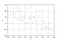Independent variable x vs. dependent variable y

To find that line `y = wx + b`, we randomly initialize two parameters: (w, b), and apply stochastic gradient descent. With a batch size of 1, i.e. updating the parameters when iterating over every data point, they gradually converge toward the optimal values (this method is also called Online SGD). Each vertical red line represents the error, i.e. the delta between the actual y value of the point and the predicted y value on the green line, also called the residual.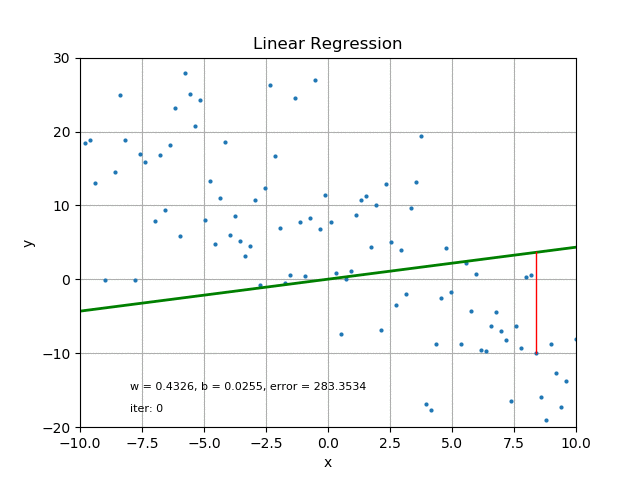Stochastic gradient descent for 2D linear regression

The goal of linear regression is to minimize MSE or Mean Squared Error, which is the mean of squares of all the red lines. We can see from above that the green line moves toward a better fit slowly and nicely. With “full” gradient descent i.e. updating parameters with the averaged gradients from all data in one iteration, the convergence is much faster.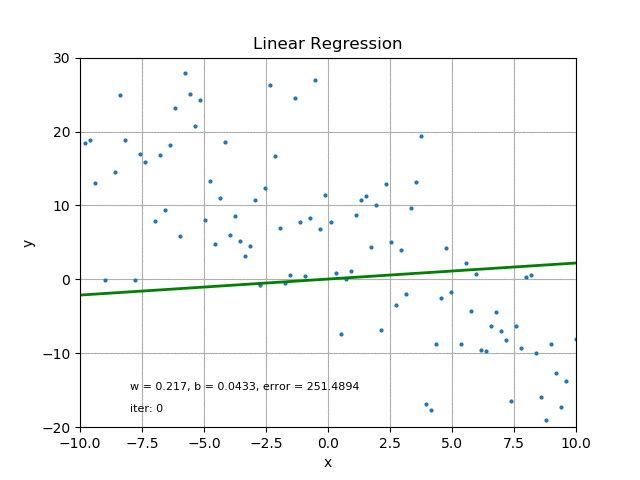Batch gradient descent

Gradient descent has an easy time for this problem because MSE for linear regression is a quadratic function of all the parameters, in this case, w and b. Therefore, it has a bowl shape, as shown below. This is called convex optimization and it’s desired whenever possible. Nonconvex optimization is much harder.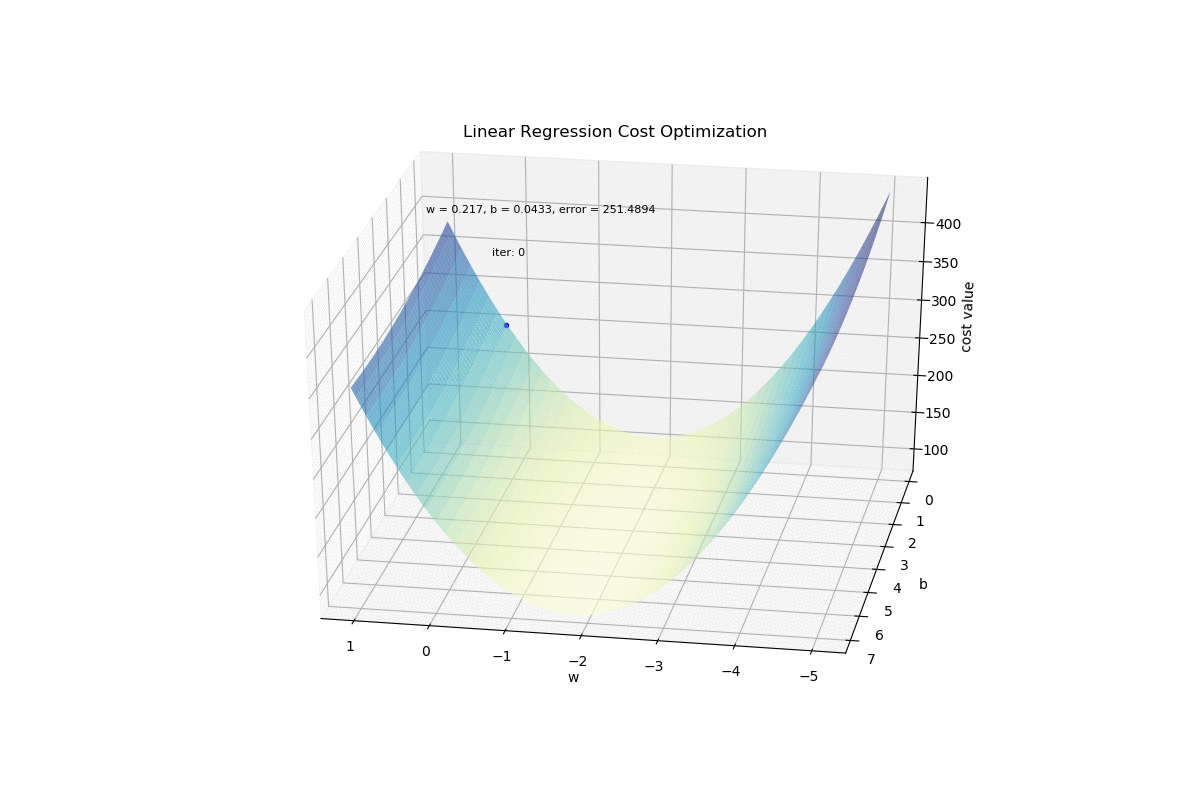Surface plot of the 2D linear regression cost function

From this plot, we can easily see that the cost is not sensitive to b but very sensitive to w. Gradient descent goes down the hill of w first because it gives more reward. It’s also intuitive in the sense that tweaking the bias isn’t as effective as tweaking the slope of the line in terms of decreasing the sum of squares of all the red lines, which is equivalent to MSE.

# Logistic Regression

Logistic regression is linear regression’s close relative. It’s called a regression but is actually a classification algorithm. Instead of computing a linear combination of the input data and parameters for real-valued outputs, it inserts the real values into the logistic sigmoid function for a number between 0 and 1. It also has a nice probabilistic interpretation for classification. (There is an infinite number of functions that map real values to (0, 1), but why pick the logistic function? The reason is quite interesting and not many classes or books talk about it. I’ll discuss it in another post.)

First, let’s look at 1D binary data for simplicity. We have a bunch of green crosses with class label 0 and some yellow dots with label 1.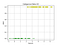1D data to be classified

The job is to come up with a vertical boundary that separates them as accurately as possible. A quick glance at the plot above shows that it’s impossible to use one dimension to perfectly separate them. Let’s see what a logistic regression would do later.

This time we are not using MSE as the loss, but something called the cross-entropy loss, or log loss.

For one data point, if its true label is 0, the loss is `-log(1-pred)`, if the true label is 1 the loss is `-log(pred)`, where `pred` is “y hat” in the equation which is the output of the sigmoid.

This may be a bit confusing but let’s look at it slowly with the drawing below. The vertical blue line is the decision boundary, meaning the algorithm predicts 1 to the right and 0 to the left.`pred` is the solid red line, i.e. the part below the curve; `1-pred` is the solid green line, i.e. the part above the curve. What the above paragraph says is that the value inside the log parentheses corresponds to the segment on the other side of the curve for each data point. The natural log is a monotonic transformation so it doesn’t affect the big and small direction of what’s inside. However, the negative sign flips the values which means the longer the solid line, the smaller the loss. For intuition, I look at the length of the dashed lines instead. Now, the longer the dashed line, the bigger the loss! And the dashed line is on the same side of the curve as the point hence much easier to look at, which is a plus!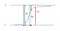log loss before the log

Warning: this is a qualitative trick I came up with and it is in no way a faithful representation of the log loss. Please have the strict definitions in mind when you actually calculate it.

Another way to look at the length of these dashed lines qualitatively is to think “prediction confidence vs. ground truth”. The goal of the log loss is this:

(1) If it’s a 1, and you predict 1 with confidence, then you are right and the loss should be small.

(2) If it’s a 1 and you predict 0 with confidence, then you are wrong and the loss should be large.

(3) If it’s a 0 and you predict 1 without much confidence, then you are moderately wrong and the loss should be moderately large.

You can check the corresponding cases in that drawing. The further away to the left or right means more confidence. The length of the dashed lines can be used as a proxy of the loss magnitude.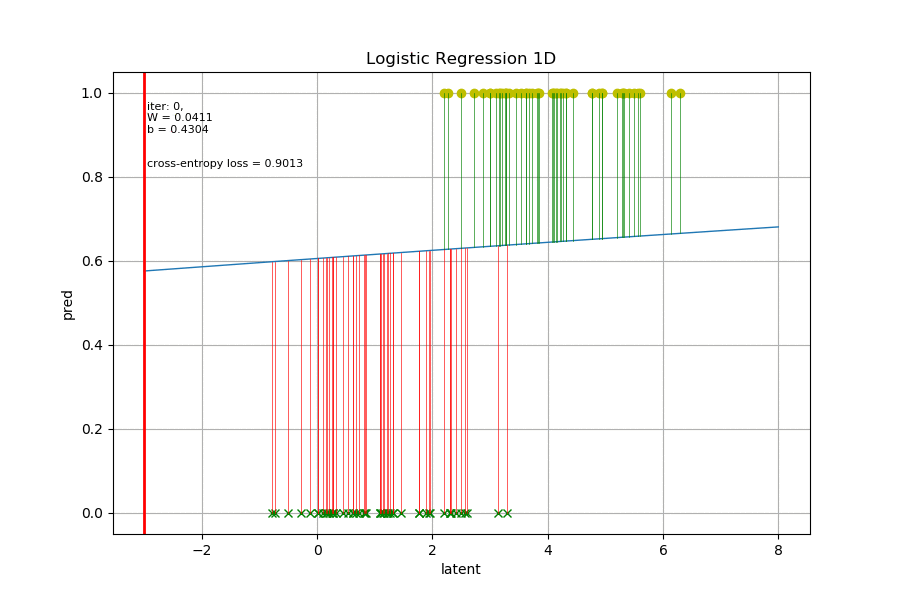Fitting a sigmoid on 1D data

In the animation above, the vertical thin lines are the delta between the sigmoid prediction and the points’ true label values, which are the same as the dashed lines in the drawing. The thick vertical red line is the decision boundary where the sigmoid outputs 0.5. I colored the delta lines red if they are wrongly classified, green if correct. In fact, the total (or mean) cross-entropy loss doesn’t care about whether they are correctly classified. The overall goal of logistic regression is to minimize those vertical segments collectively (again, its magnitude has the same monotonic direction as the log loss, it is NOT the log loss).

Now it’s more intuitive to see why that sigmoid behaved this way during the optimization. It started quite flat and in the wrong position, then it gradually moved to the optimal position despite a little bit back and forth, and it became steeper to make the segments shorter. Since there are several misclassified points, it can’t be too steep because those points will have increased loss.

This 1D example is quite a useful mental picture to understand the changing shape of the sigmoid. In higher dimensions, it becomes a hypersurface but its behavior is still similar.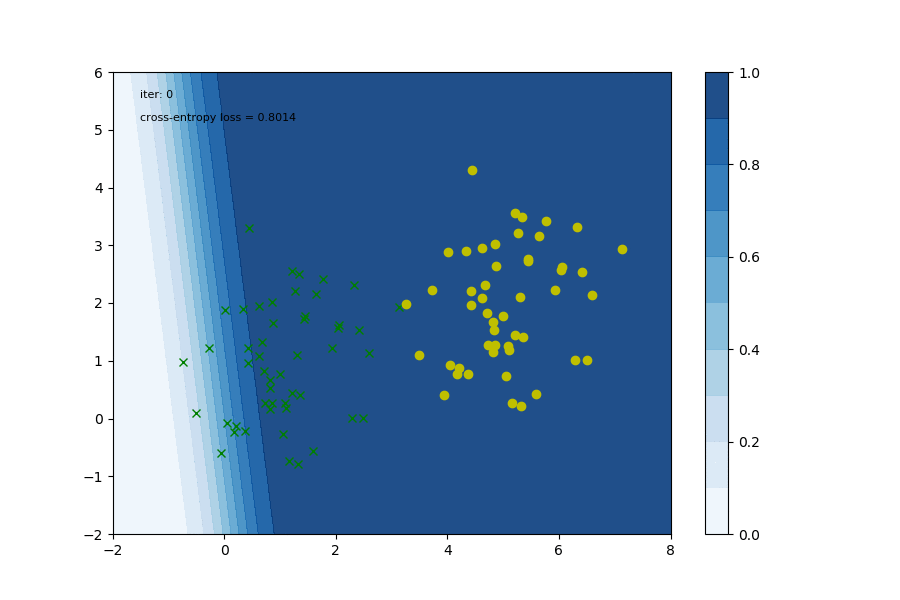Fitting a sigmoid for 2D data

This one above is a 2D dataset for binary classification. The contours represent the sigmoid surface. The behavior is the same as the 1D case, where the sigmoid moves to an optimal position for a good decision boundary and then adjusts the steepness around the boundary.

Remember that we need 3 parameters for 2D data, 2 weights and a bias. (If you implement logistic regression from scratch and forget that column of 1s for bias, it won’t converge to optimum!) The loss or cost function is a function of 3 variables, it’s not easy to visualize (3D contours in a semi-transparent 3D cube perhaps). Here I only look at the two weights vs. the loss.

We see that w1 and w2 form a nice convex surface and the path to optimum is straightforward. This is one reason why people like logistic regression.

# Summary

Linear regression and logistic regression are two of the most important and widely used models in the world. Despite their simplicity, a great number of crucial systems in various organizations rely on it on a day-to-day basis. In production, people often still pick simpler models over deep neural networks for computational performance, interpretability, and debugging purposes.

In this post, I animated the learning process in their simplest form. The “feel” I’d like to convey is how these algorithms “squash” the error (the collection of those vertical line segments) using gradient descent. When you are overwhelmed by pages of equations or large amounts of code, keep these mental pictures in mind, and don’t be intimidated. Those are just implementation details, you already understood the core concept.

In the next post of this series, I will apply similar methods to animate the learning process in neural networks using backpropagation. In another slightly math-heavy post, I will discuss the motivation for using the logistic sigmoid function from a probability perspective and talk about Generalized Linear Models. Stay tuned!

See my other posts on Medium, or follow me on Twitter.

## The Startup

Get smarter at building your thing. Join The Startup’s +800K followers.

Written by

## Logan Yang

Engineer. I write about machine learning, engineering and career. Follow me here and on Twitter for future content https://twitter.com/logancyang

## The Startup

Get smarter at building your thing. Follow to join The Startup’s +8 million monthly readers & +800K followers.

Written by

## Logan Yang

Engineer. I write about machine learning, engineering and career. Follow me here and on Twitter for future content https://twitter.com/logancyang## The Startup

Get smarter at building your thing. Follow to join The Startup’s +8 million monthly readers & +800K followers.

## More From Medium

Medium is an open platform where 170 million readers come to find insightful and dynamic thinking. Here, expert and undiscovered voices alike dive into the heart of any topic and bring new ideas to the surface. Learn more

Follow the writers, publications, and topics that matter to you, and you’ll see them on your homepage and in your inbox. Explore

If you have a story to tell, knowledge to share, or a perspective to offer — welcome home. It’s easy and free to post your thinking on any topic. Write on Medium